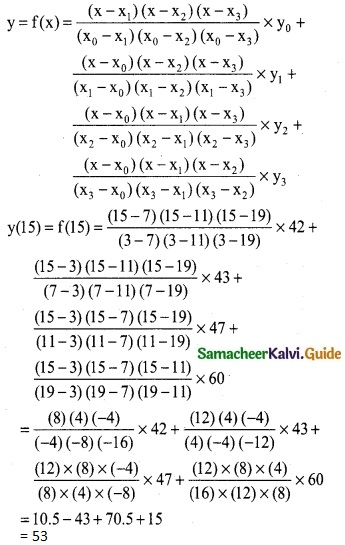Tamilnadu State Board New Syllabus Samacheer Kalvi 12th Business Maths Guide Pdf Chapter 5 Numerical Methods Ex 5.2 Text Book Back Questions and Answers, Notes.

## Tamilnadu Samacheer Kalvi 12th Business Maths Solutions Chapter 5 Numerical Methods Ex 5.2

Question 1.
Using graphic method, find the value of y when x = 48 from the following data:Solution:The value of y when x = 48 is 6.8Question 2.
The following data relates to indirect labour expenses and the level of output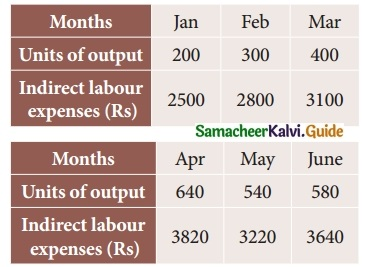Estimate the expenses at a level of output of 350 units, by using graphic method.
Solution: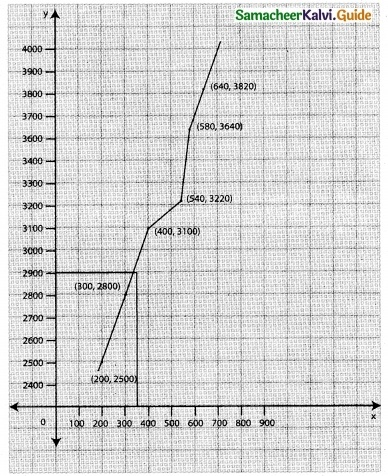Question 3.
Using Newton’s forward interpolation formula find the cubic polynomial.Solution:
Since we use the Newton’s forward interpolation formula.
y(x= x0+nh) = y0 + $$\frac { n }{1!}$$ Δy0 + $$\frac { n(n-1) }{2!}$$ Δ²y0 + $$\frac { n(n-1)(n-2) }{3!}$$ Δ³y0 + ………
To find y at x
∴ x0 + nh = x
0 + n(1) = x
∴ n = x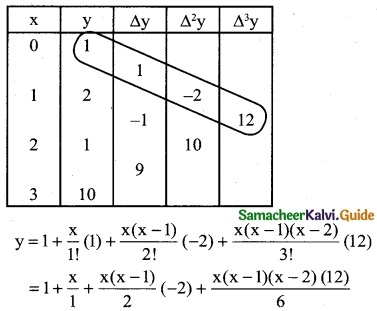= 1 + x + (x² – x) (-1) + 2x (x² – 3x + 2)
y = 1 + x – x² + x + 2x³ – 6x² + 4x
y = 2x³ – 7x² + 6x + 1
∴ f(x) = 2x³ – 7x² + 6x + 1Question 4.
The population of a city in a censes taken once in 10 years is given below. Estimate the population in the year 1955.Solution:
Let the year be x and population be y. To find the population for the year 1955.
(ie) The value of y at x = 1955
Since the value of y is required near the beginning of the table, we use the Newton’s forward interpolation formula.
y(x= x0+nh) = y0 + $$\frac { n }{1!}$$ Δy0 + $$\frac { n(n-1) }{2!}$$ Δ²y0 + $$\frac { n(n-1)(n-2) }{3!}$$ Δ³y0 + ………
To find y at x = 1955
∴ x0 + nh = 1955; x0 = 1951, h = 10
⇒ 1951 + n(10) = 1955
10n = 1955 – 1951 ⇒ 10n = 4
n = $$\frac { 4 }{10}$$ = 0.4y = 35 + 2.8 – 1.08 + 0.064
= 37.864 – 1.08
y = 36.784
∴ Population in the year 1955 is 36.784 (lakhs)Question 5.
In an examination the number of candidates who secured marks between certain interval were as follows:Estimate the number of candidates whose marks are lessthan 70.
Solution:
Since the required mark is at the end of the table, we apply Backward interpolation formula. Let the marks be x and No. of candidates be y.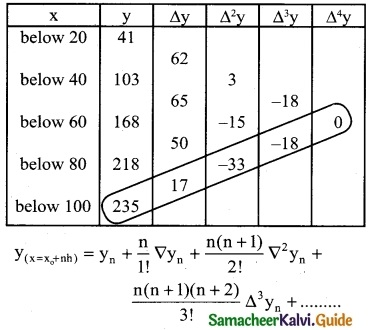To find y at x = 70
x = x0 + nh ⇒ 70 = 100 + n(20)
70 – 100 = 20n
20n = -30 ⇒ n = $$\frac { -30 }{20}$$
n = -1.5= 235 – 25.5 – 12.375 – 1.125
= 235 – 39
= 196
∴ 196 candidates secured less than 70 marks

Question 6.
Find the value of f(x) when x = 32 from the following table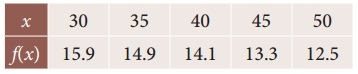Solution:
Since the value of f(x) is required near the beginning of the table, we use the Newton’s forward interpolation formula.
y(x= x0+nh) = y0 + $$\frac { n }{1!}$$ Δy0 + $$\frac { n(n-1) }{2!}$$ Δ²y0 + $$\frac { n(n-1)(n-2) }{3!}$$ Δ³y0 + ………
To find y at x = 32
∴ x0 + nh = 32;
30 + n(5) = 32
5n = 32 – 30 ⇒ 5n = 2
n = $$\frac { 2 }{5}$$
∴ n = 0.4= 15.9 – 0.4 – 0.024 – 0.0128 – 0.00832
15.9 – 0.44512 = 15.45488
= 15.45
∴ when x = 32, f(x) = 15.45Question 7.
The following data gives the melting point of a alloy of lead and zinc where ‘t’ is the temperature in degree c and p is the percentage of lead in the alloy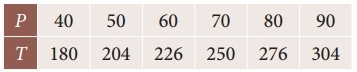Find the melting point of the alloy containing 84 percent lead.
Solution:
Since the required value is at the end of the table, apply backward interpolation formula. To find T at p = 84
T(p= p0+nh) = Tn + $$\frac { n }{1!}$$ ∇Tn + $$\frac { n(n+1) }{2!}$$ ∇²T0 + $$\frac { n(n+1)(n+2) }{3!}$$ Δ³T0 + ………
To find T at P = 84
Pn + nh = 84
90 + n(10) = 84
10n = 84 – 90
10n = -6 ⇒ n = $$\frac { -6 }{10}$$
n = -0.6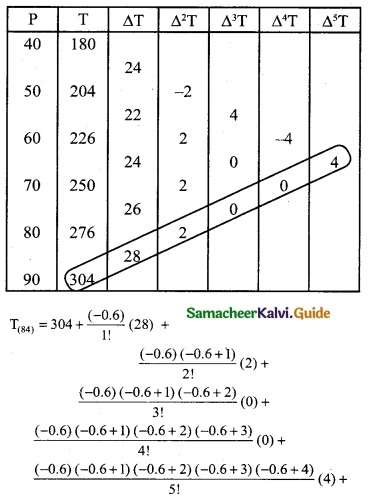= 304 – 16.8 – 0.24 – 0.091392
= 304 – 17.131392
= 286.86
Hence the melting point of the alloy is 286.86° c.Question 8.
Find f(2.8) from the followingSolution:
Since the required value is at the end of the table, apply backward interpolation formula.To find y at x = 2.8
∴ x0 + nh = 2.8
∴ 3 + n(1) = 2.8
n = 2.8 – 3
n = -0.2= 34 – 4.6 – 1.12 – 0.288
= 34 – 6.008
= 27.992
∴ f(2.8) = 27.992Question 9.
Using interpolation estimate the output of a factory in 1986 from the following dataSolution:
Here the intervals are unequal. By Lagrange’s in-terpolation formula we have,
x0 = 1974, x1 = 1978, x2 = 1982, x3 = 1990
y0 = 25, y1 = 60, y2 = 80, y3 = 170, and x = 1986.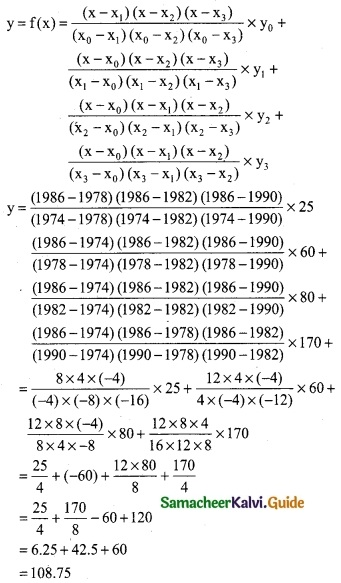∴ output in 1986 is 108.75 (thousand tones)

Question 10.
Use lagrange’s formula and estimate from the following data the number of workers getting income not exceeding Rs. 26 per month.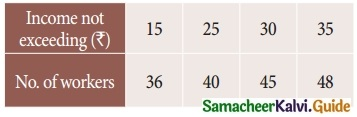Solution:
Here the intervals are unequal. By Lagrange’s In-terpolation formula we have,
x0 = 15, x1 = 25, x2 = 30, x3 = 35
y0 = 36, y1 = 40, y2 = 45, y3 = 48 and x = 26.∴ Required No.of workers = 42 Persons (approximately)Question 11.
Using interpolation estimate the business done in 1985 from the following data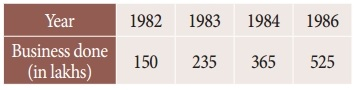Solution:
Here the intervals are unequal. By Lagrange’s formula we have,
x0 = 1982, x1 = 1983, x2 = 1984, x3 = 1986
y0 = 150, y1 = 235, y2 = 365, y3 = 525 and x = 1985.∴ Business done in the year 1985 is 481.25 lakhs.

Question 12.
Using interpolation, find the value of f(x) when x = 15Solution:
Here the intervals are unequal, By Lagrange’s in-terpolation formula we have,
x0 = 3, x1 = 7, x2 = 11, x3 = 19
y0 = 42, y1 = 43, y2 = 47, y3 = 60 and x = 15.